# Full Bridge Transformer Design: Key Aspects

## Key aspects to take into consideration the design of the transformer

The Phase Shift Full Bridge (PSFB) topology is one of the most common topologies used in the industry for designs with power levels above 500W. The transformer plays a key role in the operation of the converter and the objective of this application note is to understand the most important parameters and design trade-offs to be taken.

## 1. Leakage Inductance

The leakage inductance is a measure of how well or bad coupled is a transformer. The higher the value the worst the coupling and vice-versa. From a converter point of view the major impact of the leakage inductance is reflected in:

### Zero-Voltage-Switching (ZVS)

To achieve soft switching in the semiconductors (especially in MOSFET), the parasitic capacitance should be discharged during the dead time (DT). The energy needed for discharging this capacitance is the energy stored in the leakage inductance or series inductance as Figure 1 shows: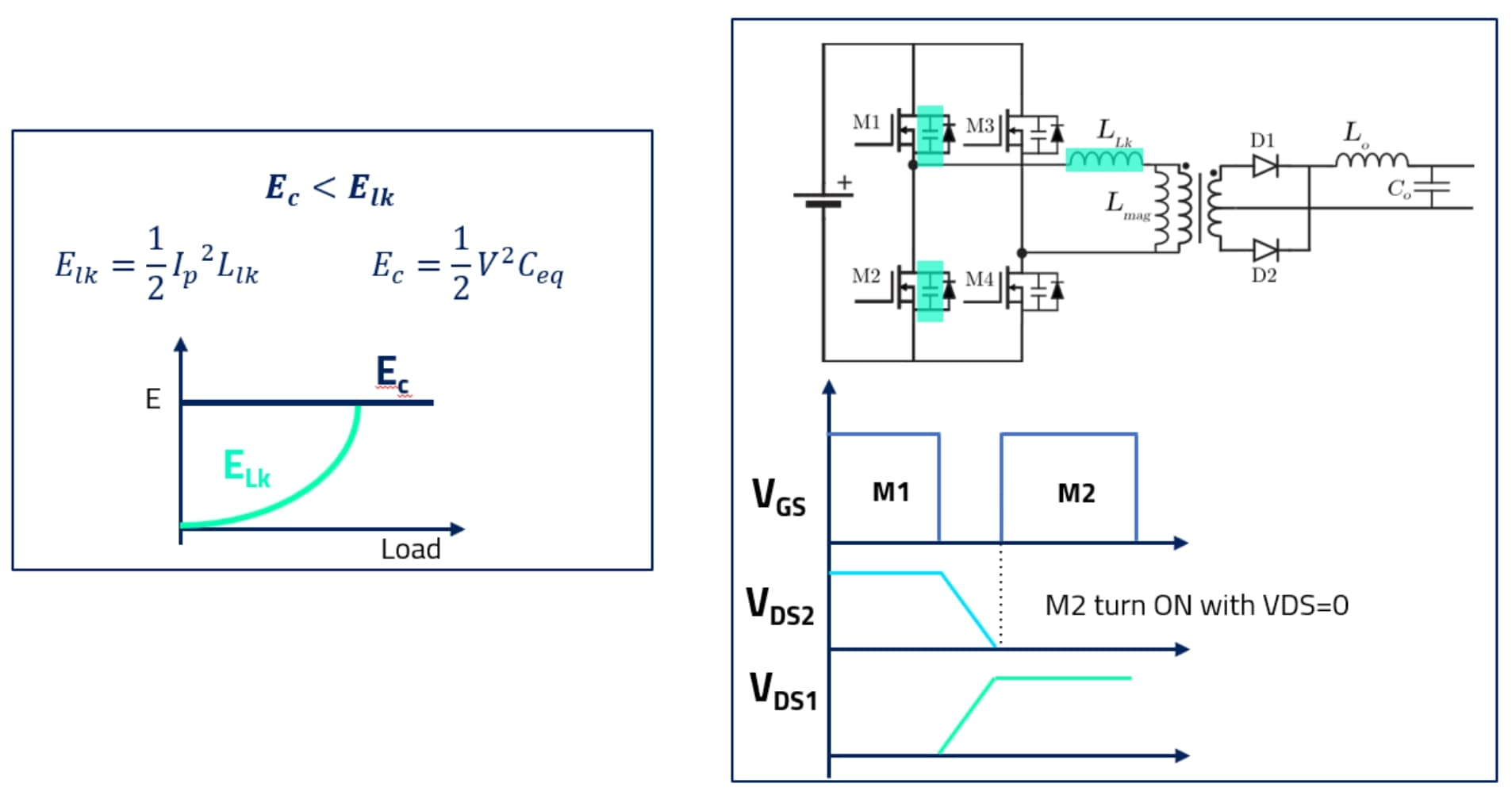Figure 1. ZVS and energy requirement in PSFB converter.

As it can be seen the energy depends on the current flowing through the transformer (commonly determined by the output load) and the value of the leakage inductance. The larger the leakage inductance value the extended the load range for ZVS but also the lower efficiency of the transformer.

### Effective Duty Cycle Loss

Including an inductance in series with an ideal transformer will affect the total energy transferred; this inductance limits the slope of the current and reduces the effective duty cycle applied to the transformer and therefore, the total energy transferred. It is quite important to take this effect into account to ensure the output voltage regulation. Figure 2 shows an example of the duty cycle loss seen primary and secondary side voltages of the transformer.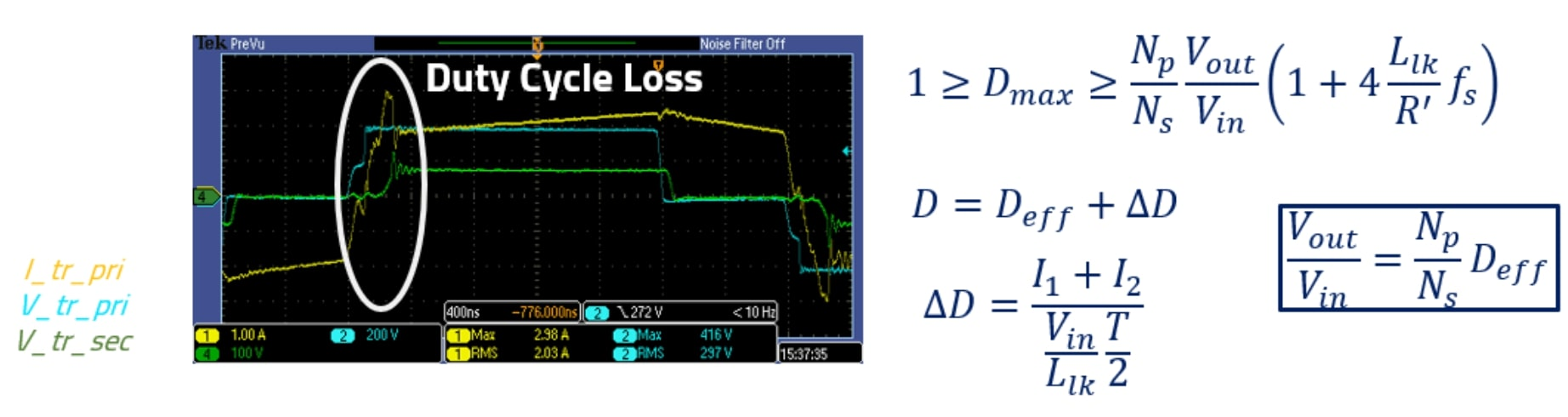Figure 2.  PSFB Duty cycle loss equation and example.

### Oscillations and voltage spike

The leakage inductance resonates with the secondary side diode capacitance and the self-winding capacitance of the transformer. Such resonance can lead to the breakdown voltage of the diodes and provoke high voltage spikes if it is not limited. This issue can be solved by minimizing the leakage inductance value or by including a clamping circuit in the primary bridge or an active snubber in the output diodes. Figure 3 shows an example of the voltage spike difference for a transformer with high and medium leakage inductance values.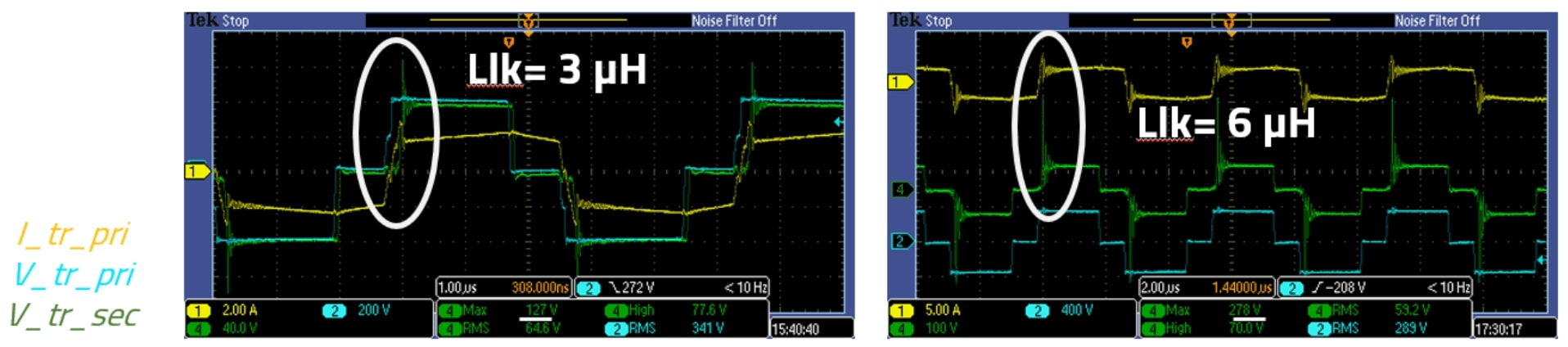Figure 3.  Voltage spike caused by leakage inductance.

From the transformer performance point of view, the leakage inductance could give a clear insight into the transformer coupling and performance. Intermediate values could be achieved by using a non-interleaved winding structure. For very high values the transformer windings shall be separated from each other in what is commonly known as a two-chamber winding. Figure 4 shows an example of the leakage inductance of three different winding arrangements.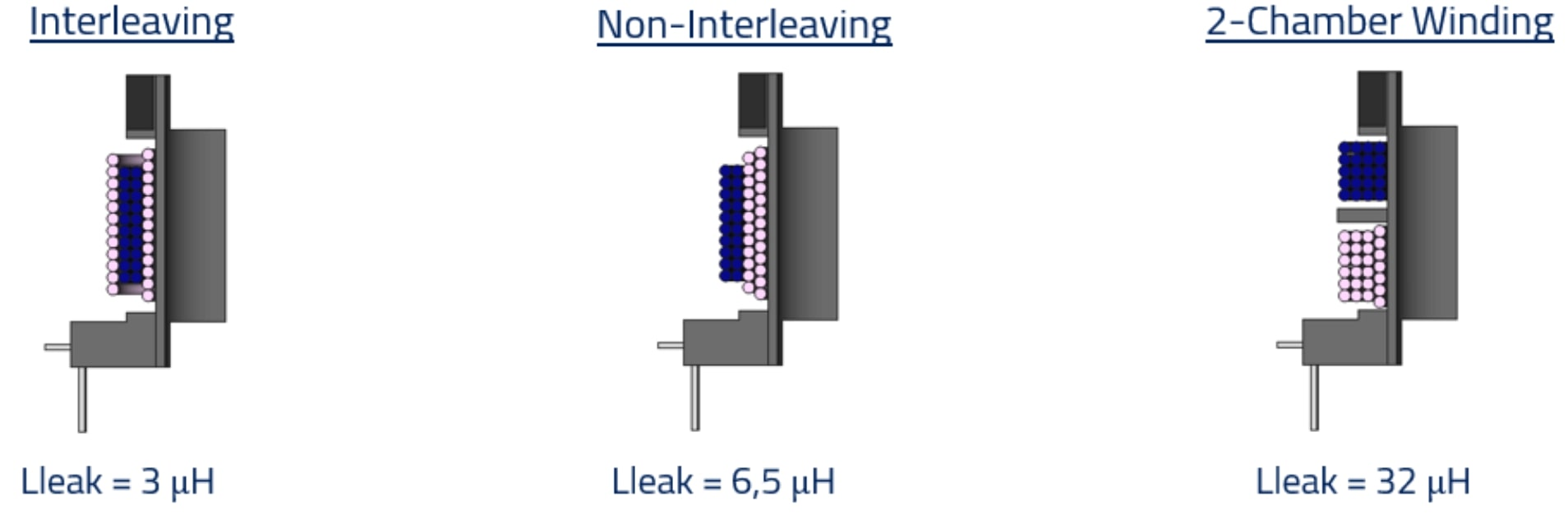Figure 4.  Leakage inductance for three different winding arrangements (pink wire: primary side; blue wire: secondary side).

As can be seen, the leakage inductance could be increased by a factor of ten by separating the wires. However, AC winding losses would be greatly affected and could lead to thermal runaway and transformer failureIt is important to note that partial interleaving (PSSP) is shown in Figure 5. A little further reduction of the leakage inductance could be achieved by using a full interleaving arrangement (PSPS) at the cost of a higher interwinding capacitance.

## 2. Turns ratio

The transformer turns ratio should be selected to ensure full power delivery at minimum input voltageDecreasing the turns ratio increases the primary current and extends the ZVS range at light loads but it also increases the reflected voltage on the secondary side rectifiers (Vsec = 2  Ns/Np Vin). Figure 5 shows an example of the primary side current level with different turns ratios.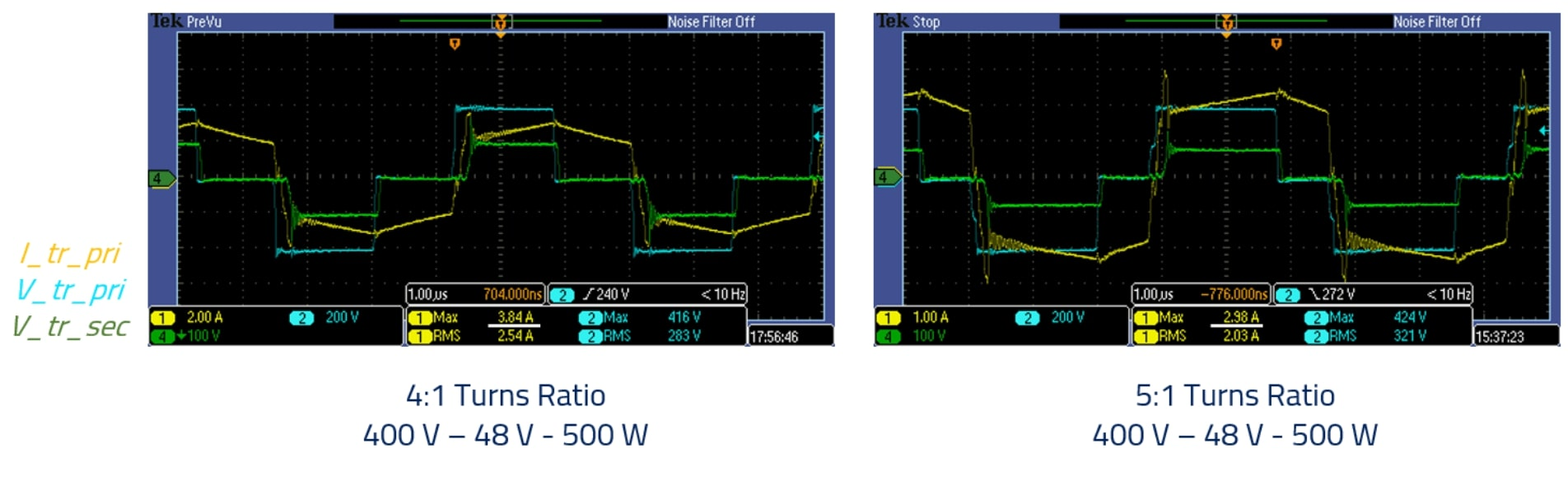Figure 5.  Transformer primary side current with different turns ratios.

## 3. Magnetizing Inductance

Three aspects should be considered in the selection of the magnetizing inductance:

### Converter control strategy.

The inductance value would be affected or imposed by the type of control used in the converter. Peak current-mode control requires high magnetizing values for minimum magnetizing currents while voltage mode control allows the use of low magnetizing inductance values.

### Inductance tolerance.

It shall be noted that high magnetizing inductance values usually rely on the ferrite core tolerance which has a typical value of ±25%, otherwise, the required number of turns in the primary side could lead to excessive conduction lossesFor reduced magnetizing values the use of gap would be needed and a tighter tolerance (±5-10%) could be achieved.

The use of magnetizing inductance (Lm) for ZVS is a strategy that usually happens naturally at low power levels, when the load main current energy stored in leakage inductance is not enough for discharging the capacitances of the semiconductors, the current through the magnetizing inductance would help since this current only depends on the voltage applied to the transformer and it is independently of the load conditionIt should be noted that such a strategy increases conduction losses, and it is only recommended for stringent light load efficiency requirements. Figure 6 shows an example of the ZVS achieved with the magnetizing inductance current.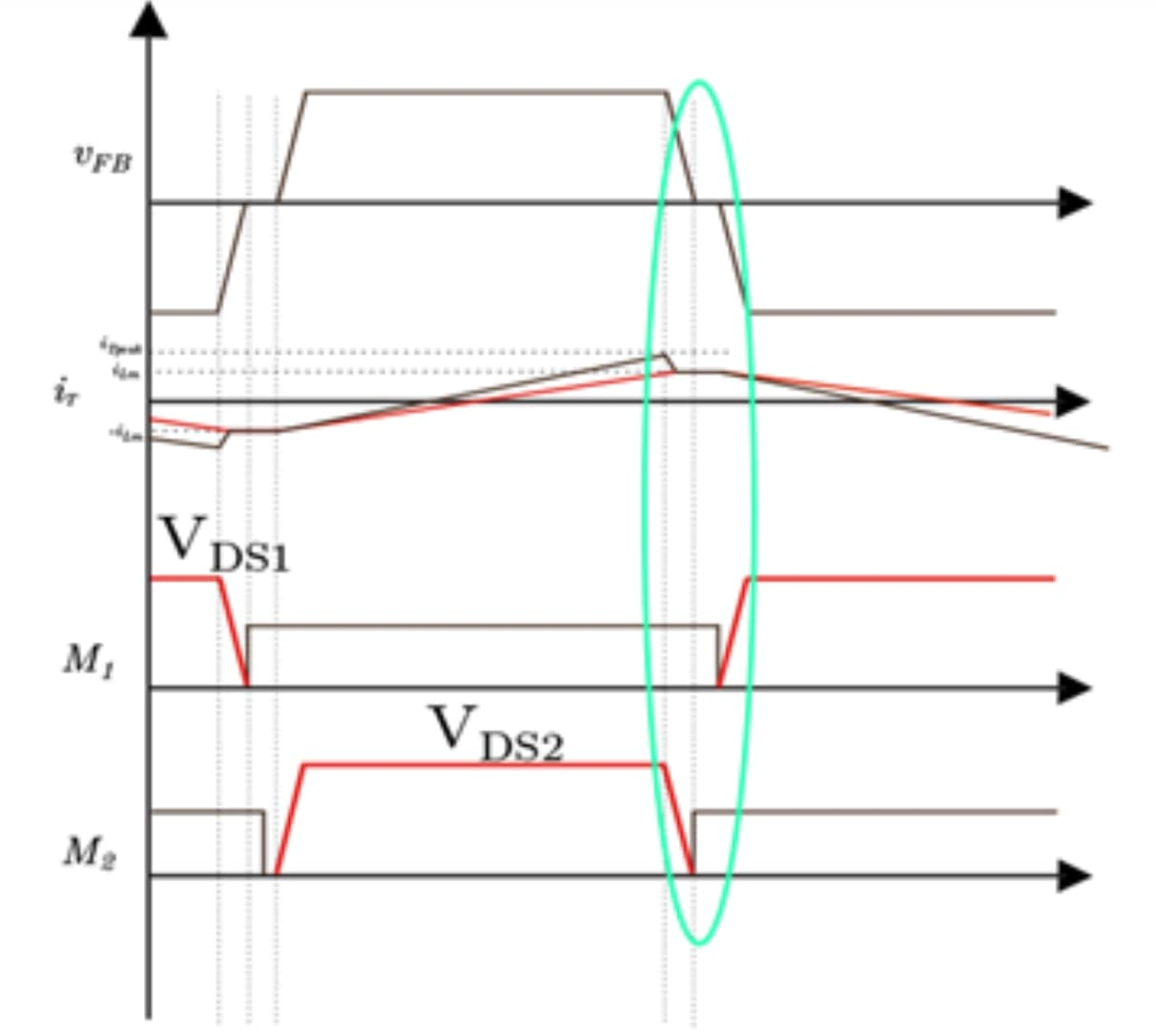Figure 6.  Transformer primary side current with different turns ratios.

## 4. Transformer Capacitances

Power converters are a source of noise that requires an input EMI filter for EMC compliance. The transformer could have a great impact on the system EMC performance since its interwinding parasitic capacitance would create a path for the common mode currents. Thus, at the design stage, its value should be considered, evaluated and if needed a faraday shield should be used between the windings. This parasitic capacitance effect should be especially considered in planar transformers where 400 pF to 1 nF interwinding capacitance could be easily found.There is another capacitance that could affect the performance of the converter and it is the self-winding capacitance of the transformer.  When a certain number of turns are wounded on a coil, a capacitance appears between each turn and therefore between the winding terminals. Such capacitance resonates with the magnetizing inductance of the transformer ending up on an impedance curve like the one seen in the figure below: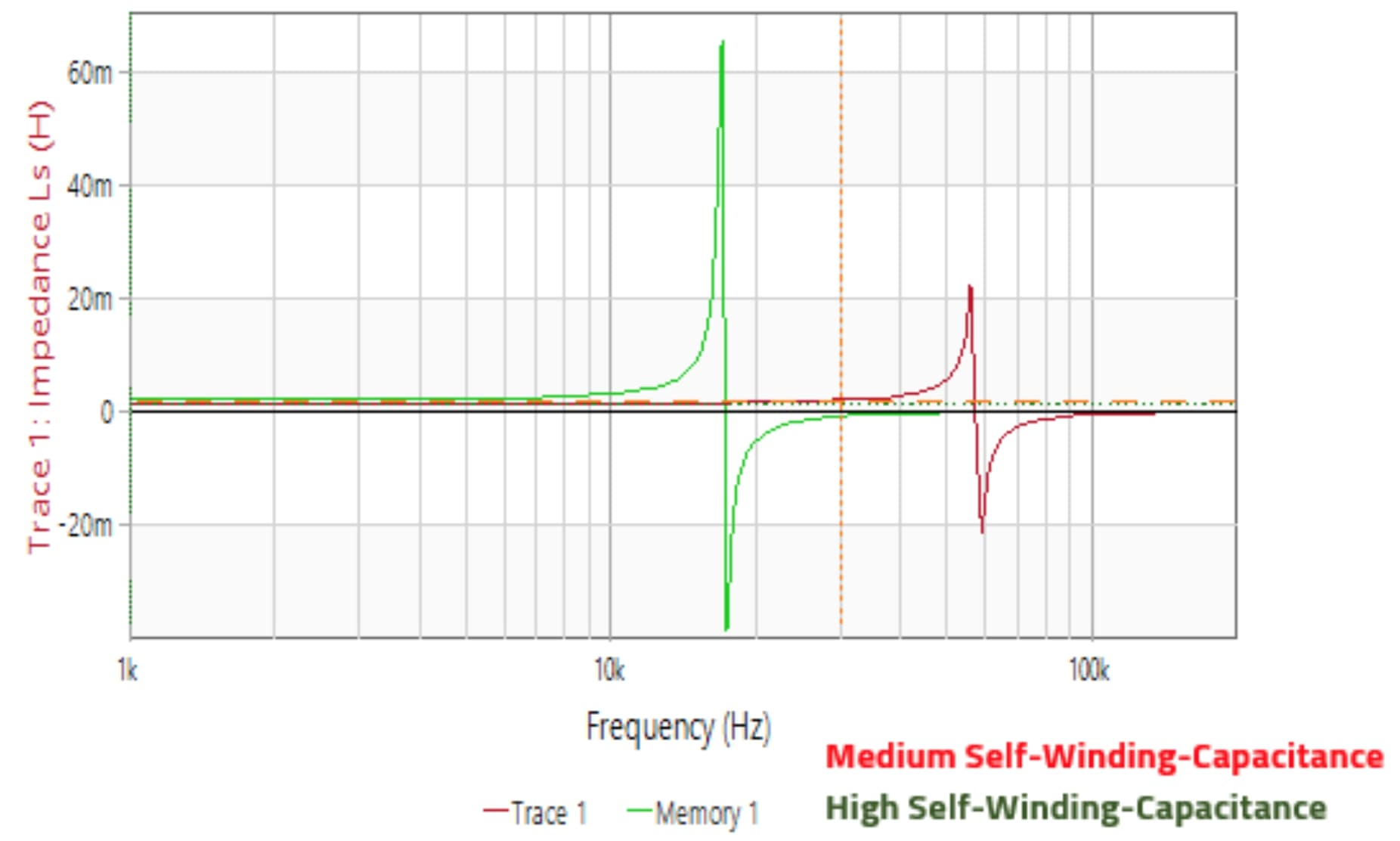Figure 7.  Impedance plot comparison of a transformer with medium and high self-winding capacitances.

The PSFB converter should be operated below the resonance frequency of the transformer where it has the desired inductive behavior.

#### Conclusions

The transformer plays a key role in the performance of the PSFB. Several parameters and trade-offs need to be considered and taken at the design stage. A good prediction of the parasitics would help in having a smooth project with low EMI noise, improve the semiconductor performance, and overall system efficiency.

Do you want the full version?